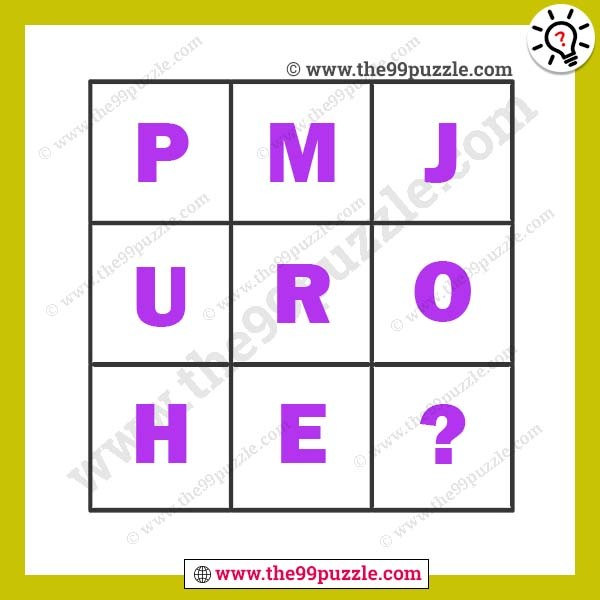# Easy letter puzzle with answer for students – Puzz194

Easy letter puzzle with answer for students. In this box letter puzzle, simply apply easy tricks to replace the question mark. Can you solve the brain-shaking letter puzzles? Here you will find many logical reasoning missing letter puzzles for students. Your challenge is to break the logical pattern used in these equations and then find the value of the missing letter which will replace the question mark. If you are good at puzzles you can find value in these easy letter puzzles.###### Explanation:

A  B  C  D  E  F  G  H  I  J  K  L  M  N  O  P  Q  R  S  T  U  V  W  X  Y  Z

In this puzzle each row, each letter difference (-3) above the alphabet series.

1st Row = P (-3) → M(-3) → J

2nd Row = U (-3) → R(-3) → O

3rd Row = H (-3) → E(-3) → B